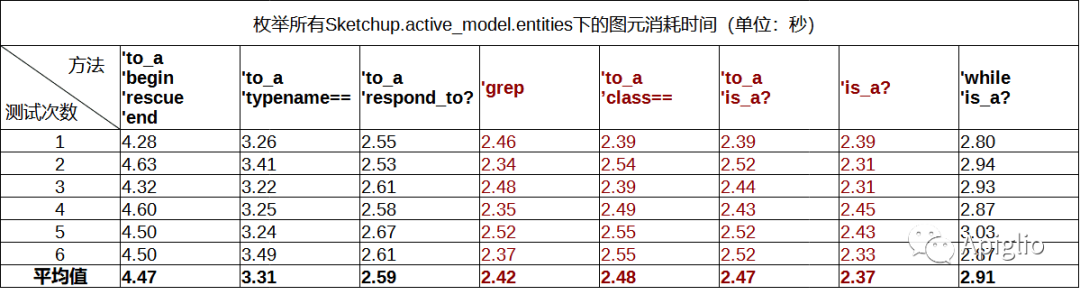# 【速度测试】SketchUp图元类型判断的速度对比

· 2022-09-17

``#令 ent 为一个图元``puts ent.typename == "Edge"``puts ent.is_a?(Sketchup::Edge)``puts ent.instance_of?(Sketchup::Edge)``puts Sketchup::Edge === ent``

``#令 ent 为一个图元``puts ent.respond_to?(:length)``puts ent.methods.include?(:length)``

``puts ent.respond_to?(:definition)``

``begin``  # 不需要判断图元类型直接执行``rescue``  # 如果报错了不会抛出异常而是执行这里的代码，这里也可以是空的``end``

``#对比class的方法``GC.start``tz=Time.now().to_f``edge_longer_than_10_meters=0``Sketchup.active_model.entities.to_a.each{|ent|``  if ent.class==Sketchup::Edge then``    if ent.length>10.m then``      edge_longer_than_10_meters+=1``    end``  end``}``puts "长度大于10米的边线图元数量：#{edge_longer_than_10_meters}"``puts "总耗时：#{Time.now().to_f-tz}秒"``#使用is_a?的方法``GC.start``tz=Time.now().to_f``edge_longer_than_10_meters=0``Sketchup.active_model.entities.to_a.each{|ent|``  if ent.is_a?(Sketchup::Edge) then``    if ent.length>10.m then``      edge_longer_than_10_meters+=1``    end``  end``}``puts "长度大于10米的边线图元数量：#{edge_longer_than_10_meters}"``puts "总耗时：#{Time.now().to_f-tz}秒"``#对比typename的方法``GC.start``tz=Time.now().to_f``edge_longer_than_10_meters=0``Sketchup.active_model.entities.to_a.each{|ent|``  if ent.typename=="Edge" then``    if ent.length>10.m then``      edge_longer_than_10_meters+=1``    end``  end``}``puts "长度大于10米的边线图元数量：#{edge_longer_than_10_meters}"``puts "总耗时：#{Time.now().to_f-tz}秒"``#使用grep的方法``GC.start``tz=Time.now().to_f``edge_longer_than_10_meters=0``Sketchup.active_model.entities.grep(Sketchup::Edge).each{|ent|``  if ent.length>10.m then``    edge_longer_than_10_meters+=1``  end``}``puts "长度大于10米的边线图元数量：#{edge_longer_than_10_meters}"``puts "总耗时：#{Time.now().to_f-tz}秒"``#判断成员的方法``GC.start``tz=Time.now().to_f``edge_longer_than_10_meters=0``Sketchup.active_model.entities.to_a.each{|ent|``  if ent.respond_to?(:length) then``    if ent.length>10.m then``      edge_longer_than_10_meters+=1``    end``  end``}``puts "长度大于10米的边线图元数量：#{edge_longer_than_10_meters}"``puts "总耗时：#{Time.now().to_f-tz}秒"``#不使用to_a的is_a?方法``GC.start``tz=Time.now().to_f``edge_longer_than_10_meters=0``Sketchup.active_model.entities.each{|ent|``  if ent.is_a?(Sketchup::Edge) then``    if ent.length>10.m then``      edge_longer_than_10_meters+=1``    end``  end``}``puts "长度大于10米的边线图元数量：#{edge_longer_than_10_meters}"``puts "总耗时：#{Time.now().to_f-tz}秒"``#处理异常的方法``GC.start``tz=Time.now().to_f``edge_longer_than_10_meters=0``Sketchup.active_model.entities.to_a.each{|ent|``  begin``    if ent.length>10.m then``      edge_longer_than_10_meters+=1``    end``  rescue``  end``}``puts "长度大于10米的边线图元数量：#{edge_longer_than_10_meters}"``puts "总耗时：#{Time.now().to_f-tz}秒"``#使用while的方法``GC.start``tz=Time.now().to_f``edge_longer_than_10_meters=0``index=0``len=Sketchup.active_model.entities.length``while index<len do``  ent=Sketchup.active_model.entities[index]``  if ent.is_a?(Sketchup::Edge) then``    if ent.length>10.m then``      edge_longer_than_10_meters+=1``    end``  end``  index+=1``end``puts "长度大于10米的边线图元数量：#{edge_longer_than_10_meters}"``puts "总耗时：#{Time.now().to_f-tz}秒"``（1）使用ruby对象类型的判断方法要比字符串判断方法节约30%左右的时间。

（2）在不涉及修改图元的情况下使用 while 语句非但没有提速，反而速度较慢，因次没有必要用它替代迭代器。

（3）判断是否拥有成员的 respond_to? 方法速度也与判断类型相近，因此对于上文所说的群组组件的判断，用此方法更为理想。

（4）有隔离影响功能的 to_a 方法会额外增加一小部分执行时间，因此使用 grep 方法既可以获得较高的执行效率，同时还可以做到隔离影响，是相对而言最合理的方法。

（5）处理异常的 rescue 语句，速度低于字符串的比较，这是毫不意外的，实现具体功能时还是应该尽量避免本身可控的不确定性。

（完）